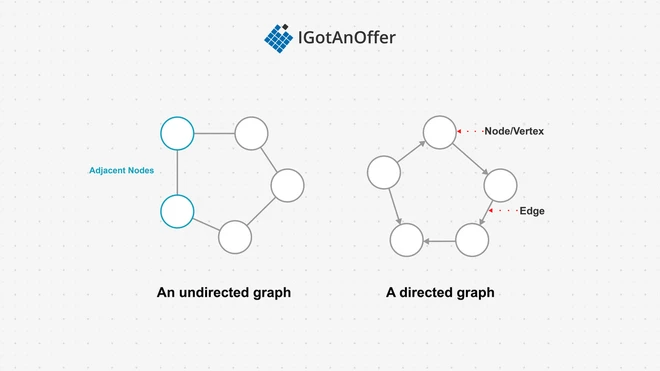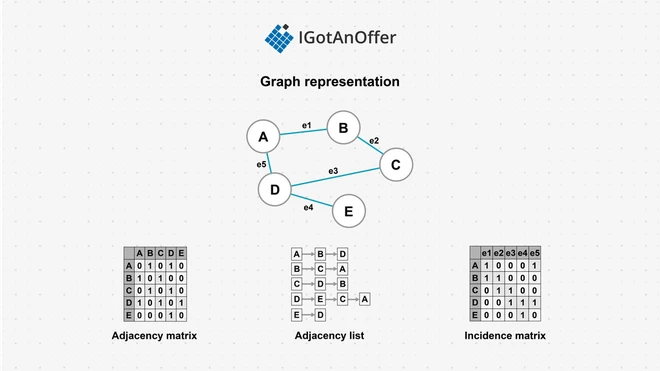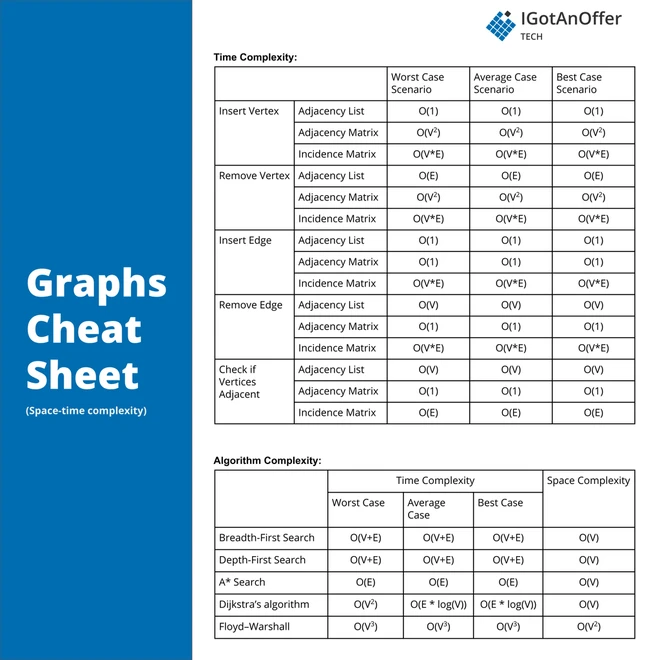# 50+ graph interview questions and cheat sheetTo ace your coding interview for a software engineering job, you’ll need to understand graphs. They come up frequently in coding interviews and are fundamental to many other data structures too.

Let’s take a look at some typical graph questions.

7 typical graph interview questions

• Given a reference of a node in a connected undirected graph, return a deep copy (clone) of the graph.
• Given an undirected graph, determine if it contains a cycle.
• Implement breadth-first search on a graph.
• Given a start word, an end word, and a dictionary of valid words, find the shortest transformation sequence from start to end such that only one letter is changed at each step of the sequence, and each transformed word exists in the dictionary.
• Given a directed graph, return true if the given graph contains at least one cycle, else return false.
• There are a total of numCourses courses you have to take, labeled from 0 to numCourses-1. You are given an array of prerequisites, where prerequisites[i] = [Ai , Bi] indicates that you must take course Bi first if you want to take course Ai. Determine if you can finish all courses.
• Find the shortest path between two nodes u and v, with path length measured by the number of edges.

Below, we take a look at some more questions and provide you with links to high quality solutions to them. We explain how graphs work, their variations, and the most important things you need to know about them, including a useful "cheat sheet" to remind you of the key points at a glance.

This is an overview of what we’ll cover:

## 1. Easy graph interview questions

You might be tempted to try to read all of the possible questions and memorize the solutions, but this is not feasible. Interviewers will always try to find new questions, or ones that are not available online. Instead, you should use these questions to practice the fundamental concepts of graphs.

As you consider each question, try to replicate the conditions you’ll encounter in your interview. Begin by writing your own solution without external resources in a fixed amount of time.

If you get stuck, go ahead and look at the solutions, but then try the next one alone again. Don’t get stuck in a loop of reading as many solutions as possible! We’ve analysed dozens of questions and selected ones that are commonly asked and have clear and high quality answers.

Here are some of the easiest questions you might get asked in a coding interview. These questions are often asked during the "phone screen" stage, so you should be comfortable answering them without being able to write code or use a whiteboard.

## 2. Medium graph interview questions

Here are some moderate-level questions that are often asked in a video call or onsite interview. You should be prepared to write code or sketch out the solutions on a whiteboard if asked.

## 3. Hard graph interview questions

Similar to the medium section, these more difficult questions may be asked in an onsite interview or a video call. You will likely be given more time if you are expected to create a full solution.

## 4. Graph basics

In order to crack the questions above and others like them, you’ll need to have a strong understanding of graphs, how they work, and when to use them. Let’s get into it.

### 4.1 What are graphs?

A graph is an abstract data structure represented by vertices connected by edges. Vertices are also known as nodes. Vertices sharing an edge are known as adjacent.

In directed graphs, sometimes called digraphs, the edges between nodes have a direction attribute to show which way a relationship between two nodes goes. Non-directed graph edges have no direction, meaning the relationship goes in both directions.

A loop is an edge from a vertex back to the same vertex. Simple graphs do not have any loops, nor do they have multiple edges between vertices. Most graph problems and graph algorithms deal with simple graphs.Edges can be weighted or have an edge value, relating to an attribute such as capacity, length, cost, time, etc. Neural networks are examples of graphs with edge values, called weights.

Traversing a graph, or graph search, involves visiting each vertex in a graph. Because graphs are not hierarchical like trees, a list of visited nodes needs to be kept when traversing a graph to ensure previously visited nodes are not revisited.

There are two main traversal strategies, namely depth-first search (DFS), and breadth-first search (BFS).

The objective of a depth-first search is to explore deeper in a graph at each move. Deeper means further from the starting, or source, vertex as possible on each navigation move. This is often done using recursion. The general method is:

• Visit vertex “s”
• Mark the vertex as visited
• Visit each unvisited vertex with an edge to “s” recursively

The objective of a breadth-first search is to explore all the vertices adjacent to the starting vertex, before moving on to vertices further from the starting vertex. This is often done by queuing all adjacent vertices and visiting them. As a vertex is visited, its unvisited adjacent vertices are added to the end of the queue. The general method is:

• Visit vertex “s”
• Mark “s” as visited
• Add each of the vertices adjacent to “s” to the queue
• Dequeue the next vertex and repeat

Graph traversal is often used to find a path from one vertex to another. This is useful in many applications, such as network route finding, transport routing, and decision planning, among many others.

When finding a path in a graph, we are often interested in finding the shortest path. The shortest path is defined as the path that has the lowest cost. The cost of a path is the sum of all the edge weights along the path.

Dijkstra's algorithm is a common method used to find the shortest path between two vertices. The algorithm finds the path with the lowest cost from a starting or source vertex “S” to an end vertex “E.” It does this by starting from the source vertex “S,” and calculating and recording the cost to each of the adjacent vertices to “S.” “S” is now considered processed. The algorithm is then repeated on the vertex with the lowest cost from the remaining vertices. If the cost to a particular vertex is less than the cost currently recorded for that vertex, the cost is updated to the lower cost. This repeats until the end vertex “E” is reached, and all the costs from nodes adjacent to “E” have been calculated.

Implementing Dijkstra's algorithm is usually done using a priority queue and a set. The priority queue contains all the vertices which have not yet been fully processed. The set holds all the vertices that have been fully explored. When popping from the priority queue to get the next vertex to process, the vertex with the lowest current cost is returned. To prime the algorithm, the cost value for every vertex is set to infinity, except for the starting vertex, which is set to 0.

Dijkstra's algorithm requires that all weights are non-negative. Dijkstra's algorithm can also be run without a specific end vertex. In this case, the cost of all paths from a source vertex to any other vertex can be calculated and stored.

#### 4.1.1 Types of graphs (Java, Python, C++)

Most languages do not have directly usable graph implementations, like arrays or linked lists. Generally trees are implemented based on the particular use case.

#### 4.1.2 How graphs store data

There are many different ways to represent and store graphs.

An adjacency matrix can be used, where the vertices are listed as the columns and rows indices, and the values are numbers representing if there is an edge (and what weight the edge is) between the vertices. One issue with an adjacency matrix is that it takes a large amount of space, O(N^2) to store the graph.

The adjacency list is another common implementation, which uses less space than the matrix. An adjacency list can be thought of as an array of linked lists. Each element in the array is a reference to the head of a linked list, one per vertex in the graph. The linked list contains all vertices adjacent to that starting vertex.An incidence matrix is another way to represent a graph. In this matrix, the columns are the edges, and the rows are the vertices. The values of the matrix indicate if there is a connection, or incidence, between the corresponding vertex and edge. Because any particular edge can only have two vertices connected to it, the incidence matrix has the property that all column sums equal two. This can be used to verify an incidence matrix.

#### 4.1.3 How graphs compare to other data structures

Graphs can be thought of as the superset of many other structures in computer science, for example trees, heaps, linked-lists, neural networks, and so on.

## 5. Graphs cheat sheet### 5.2 Cheat sheet explained

The cheat sheet above is a summary of information you might need to know for an interview, but it’s usually not enough to simply memorize it. Instead, aim to understand each result so that you can give the answer in context.

The cheat sheet is broken into time complexity (the processing time for various graph operations) and algorithm complexity (the amount of time and space used for common algorithms).

#### 5.2.1 Time complexity

The time complexity for various graph operations depends on the type of implementation used for the graph.

An adjacency list performs well on inserting new vertices, and inserting new edges (links between vertices), as inserting into a linked list is constant time, and adding the new vertex to the adjacency list's array is amortized constant time. Removing a vertex is a factor of the number of edges the vertex has, as it also needs to be removed from all the lists of its connected vertices. Checking if vertices are adjacent requires reading and checking each element of the linked lists for the two vertices. This can take up to O(V) time if the vertices are fully connected to all other vertices.

Adjacency matrices perform well on querying vertex adjacency, as this is an index lookup on the two vertices in the matrix. This is a constant time operation, which is a large advantage over the adjacency list. Adjacency matrices have a similar performance advantage when removing an edge, for the same reason. Inserting a new vertex into an adjacency matrix can be very expensive, in quadratic time, as a new matrix may need to be created and all elements copied. This can be reduced if the underlying matrix is initially made larger than required, however this requires even more space usage. This can be significant, as the space requirement for the unused matrix is quadratic. Removing a vertex suffers the same time inefficiency, as the entire matrix needs to be re-written.

Incidence matrices are more efficient than adjacency matrices on many dimensions if the number of vertices is much greater than the number of edges. If the number of edges and vertices are similar, then an incidence matrix will be similar to the quadratic times of an adjacency matrix.

#### 5.2.2 Space complexity

The storage or space needed for a graph also depends on the implementation used, and the ratio of vertices to edges. For fully connected graphs, the space used for both matrix and list representations converge. Adjacency lists are more space efficient for graphs with far fewer edges than vertices. Incidence matrices can also be more efficient than adjacency matrices, but less so than lists, if there are fewer edges than vertices.

#### 5.2.3 Graph algorithms complexity

Both depth-first and breadth-first search require visiting each vertex along each edge, and therefore take O(V+E) time. Depth-first search requires a stack to keep track of the vertices to visit; breadth-first requires a queue. In either case, these structures may need to store at least a subset of all the graph’s vertices, requiring O(V) space.

Dijkstra's algorithm requires a priority queue of all unprocessed vertices, and a set containing all processed vertices, therefore using O(V) space. The time that Dijkstra's algorithm runs in is similar to a breadth-first search, plus a component needed to maintain the priority queue for unprocessed nodes. If the priority queue is implemented using a binary tree as its base, this can add O(log V) time per search. As we need to search for each edge, it becomes O(E*log V). If a simple array is used instead of the priority queue or similar structure, each search for the next vertex for each edge can result in O(V*E), with O(V^2) time if the number of edges and vertices are similar.

A* search is a more time-efficient version of Dijkstra's algorithm, that uses heuristics to guide the search path more effectively. This can shorten the search time to the order of the number of edges in the graph.

Floyd–Warshall is an algorithm to find the shortest distances between all pairs of vertices in a graph (as opposed to finding the shortest path between one pair, as for Dijkstra). It can achieve this in O(v^3) time, i.e. it is not dependent on the number of edge connections in the graph.

## 6. Mock interviews for software engineers

Before you start practicing interviews, you’ll want to make sure you have a strong understanding of not only graphs but also the rest of the relevant data structures. Check out our guides for questions, explanations and helpful cheat sheets.

Once you’re confident in all of the data structures, you’ll want to start practicing answering coding questions in an interview situation. One way of doing this is by practicing out loud, which is a very underrated way of preparing. However, sooner or later you’re probably going to want some expert interventions and feedback to really improve your interview skills.

That’s why we recommend practicing with ex-interviewers from top tech companies. If you know a software engineer who has experience running interviews at a big tech company, then that's fantastic. But for most of us, it's tough to find the right connections to make this happen. And it might also be difficult to practice multiple hours with that person unless you know them really well.

Here's the good news. We've already made the connections for you. We’ve created a coaching service where you can practice 1-on-1 with ex-interviewers from leading tech companies. Learn more and start scheduling sessions today.